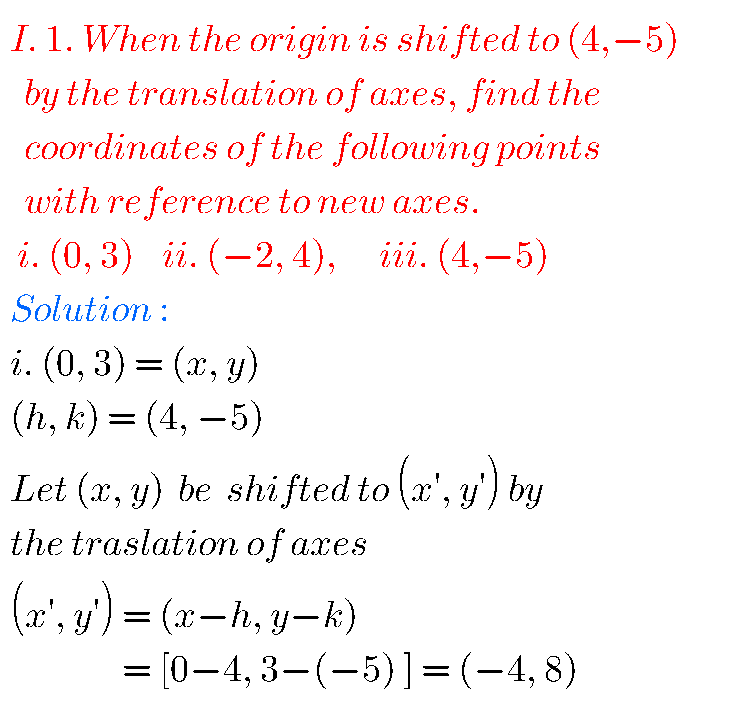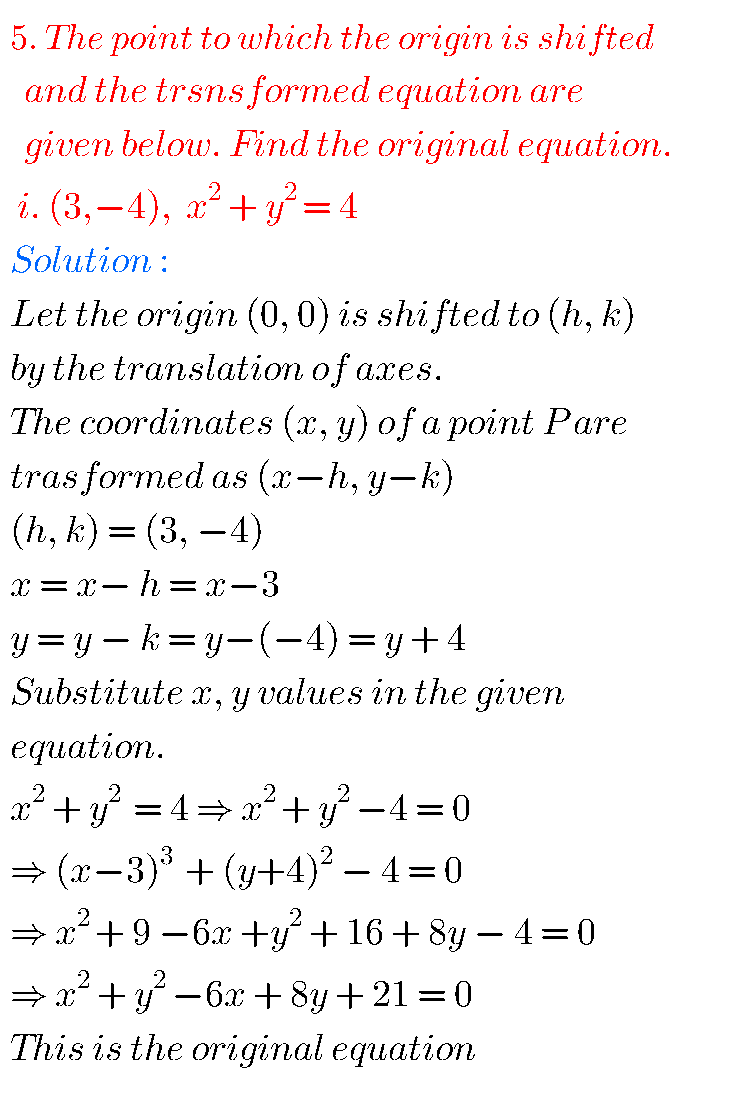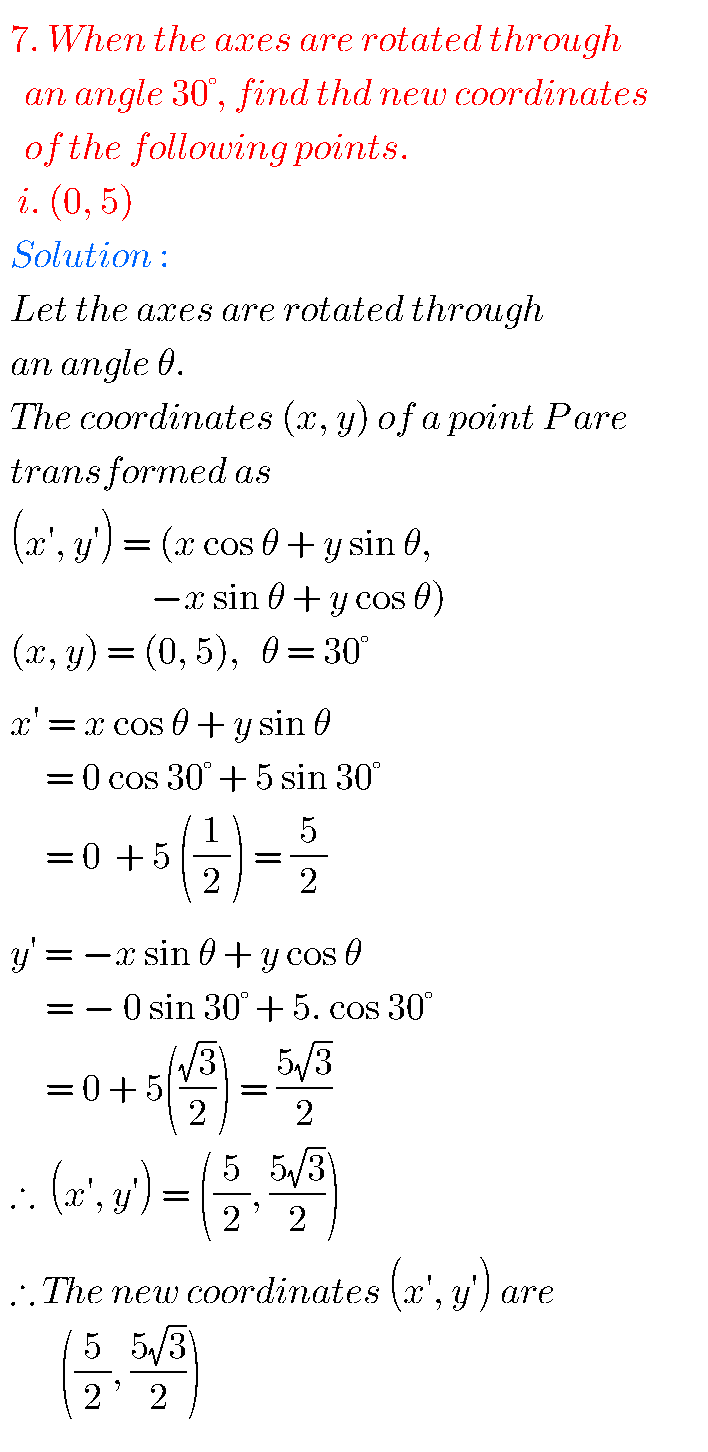# Inter Maths solutions for Transformation of Axes Exercise 2(a)

Inter Maths 1B text book Transformation of Axes Exercise 2(a) are given.

These solutions are very easy to understand. Stydy the text book lesson Transformation of Axes very well.

Observe the given example problems and solutions in the text book. Try them.

Observe the given solutions and try them in your own method.

You can also see

Inter Maths 1A textbook solutions

Inter Maths 1B textbook solutions

Inter Maths IIA textbook solutions

Inter Maths IIB textbook solutions

Nios Solutions for Maths 311 Book 1 Sets 1.1

## Inter Maths 1B solutions for Transformation of Axes Exercise 2(a)

Exercise 2(a)## Maths solutions for Transformation of Axes Exercise 2(a)### Transformation of Axes solutions Inter exercise 2(a)Note : Observe the solutions and try them in your own method.SSC Maths solutions

Ncert solutions for maths class 6 chapter 14